# Find Arc Length Calculations for Calculus with Wolfram|Alpha

April 4, 2012 —Comments Off

One of the features of calculus is the ability to determine the arc length or surface area of a curve or surface. An arc length is the length of the curve if it were “rectified,” or pulled out into a straight line. You can also think of it as the distance you would travel if you went from one point to another along a curve, rather than directly along a straight line between the points.

To see why this is useful, think of how much cable you would need to hang a suspension bridge. The shape in which a cable hangs by itself is called a “catenary,” but with a flat weight like a roadway hanging from it, it takes the shape of a more familiar curve: a parabola.The Golden Gate Bridge, shown above, has a main span of 4,200 feet and two main cables that hang down 500 feet from the top of each tower to the roadway in the middle. From this information we can use Wolfram|Alpha to find the equation defining the parabolic curve of the cables: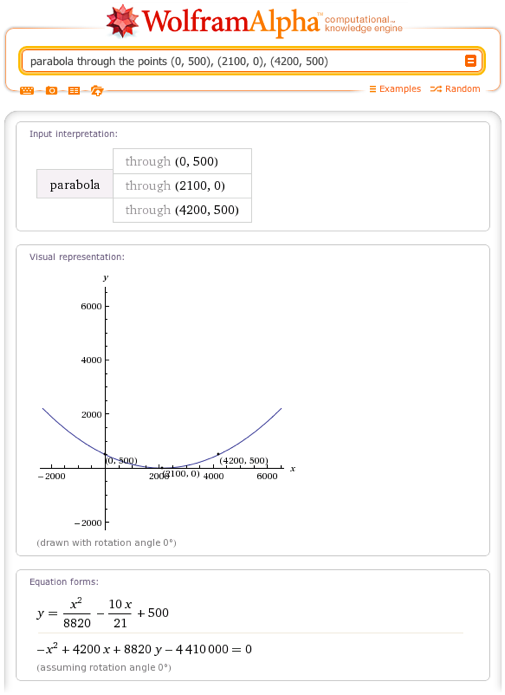Entering the equation from the “Equation forms” pod into the input box, we now ask Wolfram|Alpha for the length of each cable over the main span: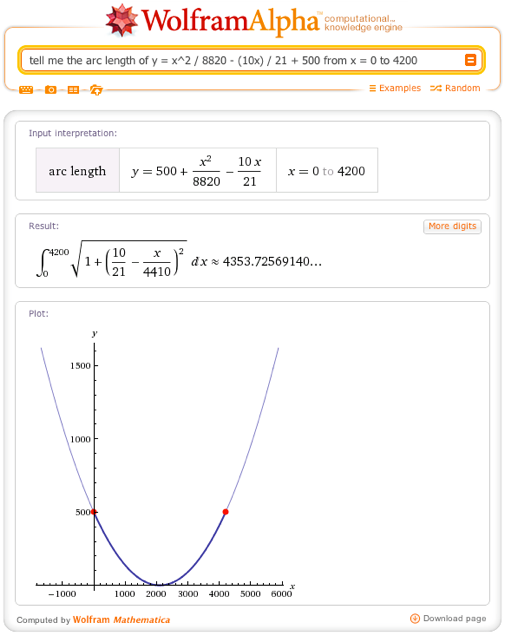So the main part of each cable is about 4,354 feet long—slightly more than the distance between the towers. Notice that Wolfram|Alpha shows the calculation needed to find the arc length (just like finding an area under a curve, integration is required) as well as the answer. When possible, Wolfram|Alpha returns an exact answer; in this case the answer involves the hyperbolic sine function, which you can then have Wolfram|Alpha approximate to any desired accuracy using the More digits button on the right.

What about curves in three or more dimensions? One common exercise in a standard calculus course is to find the arc length of a helix. This could be the length of wire needed to form a spring or the amount of tape needed to wrap a cylinder without leaving any gaps.

A helix can be expressed as a parametric curve in which the x and y coordinates define a circle, while the z coordinate increases linearly. For example: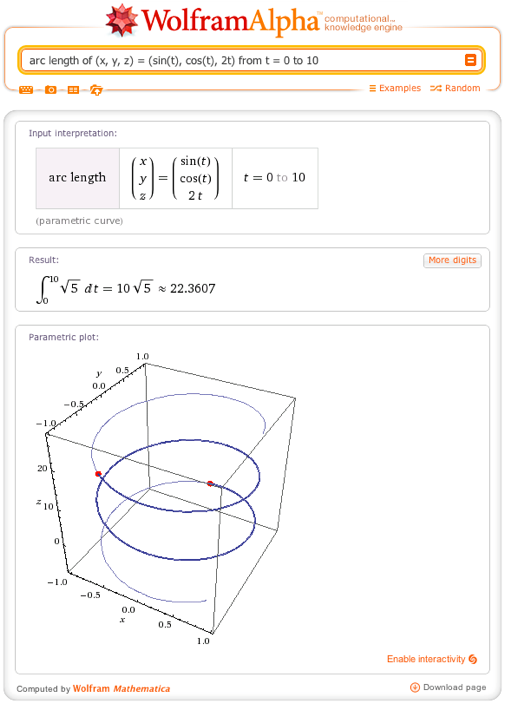You can also find arc lengths of curves in polar coordinates. In the example below, using the variables r and θ causes Wolfram|Alpha to guess that the given equation was intended to be graphed in polar coordinates: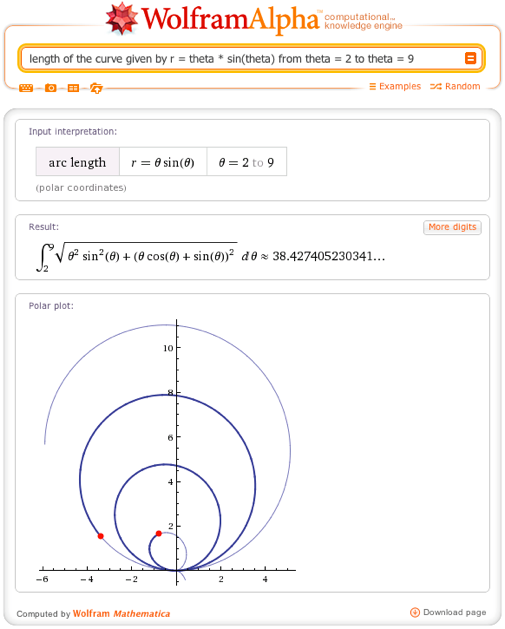Finally, you do not always have to specify a curve and two endpoints to explore arc lengths using Wolfram|Alpha. If you don’t, Wolfram|Alpha will produce a calculator in which you can dynamically change the values you did not specify.

For example, suppose you wanted to find out how far a ball travels when thrown at an angle of 45° with an initial velocity of 50 meters per second. Entering just the equations of motion for the ball (using a little Newtonian physics) and the starting point (t = 0), you can see how the distance changes as you change the second value for t: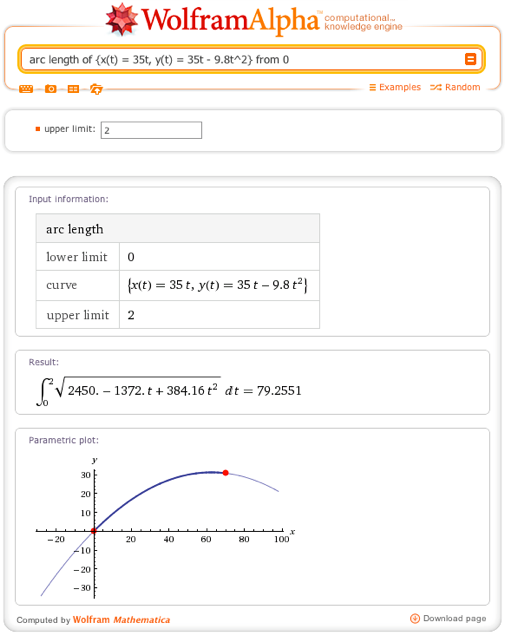In the image above, Wolfram|Alpha has chosen a value of 2 for the second value of t. Changing this 2 to 3.5 causes the result and the image to change: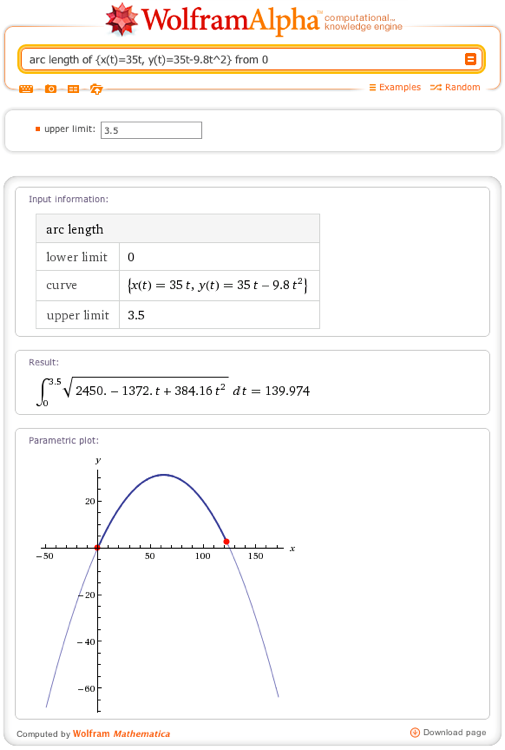We hope you enjoy this new feature, and are looking forward to bringing you more fun and useful math functionality.

Nice capability!

Except I thought that cables hanging in suspension formed a catenary y = a cosh(x/a) rather than a parabola y = ax^2+b

Can WA handle this?

Posted by Kim April 4, 2012 at 7:07 pm

No Kim, a free hanging cable is a catenary which W|A (unfortunately) cannot handle, at least as far as I can tell. Parabolas occur when there is an even load distribution across the length of the cable so that the weight of the cable is essentially zero compared to the load.

Posted by Reginald April 8, 2012 at 11:06 am

It is relatively straight forward to calculate the arc length of a catenary described by the function y = a cosh(x/a). Generally the arc length, s, of a real function f(x), with a derivative f'(x), that is continuous on [-b,b] can be found as

s = Integrate[Srqt[1+f'(x)^2],{x,-b,b}]

For this particular example, the arc length of the function y = a cosh(x/a) on [-b,b] can be determined by

s = Integrate[Sqrt[1 + (D[a*Cosh[x/a], x])^2], {x, -b, b}]

or

s = Integrate[Sqrt[1 + (Sinh[x/a])^2], {x, -b, b}]

Evaluating this integral yields the following result

s = 2 a Sqrt[Cosh[b/a]^2] Tanh[b/a]

This result can be numerically evaluated for any particular a \[Element] (0, \[Infinity]) and b \[Element] [0, \[Infinity]).

Posted by Ben April 26, 2015 at 1:55 pm

A wonderful article. Very interesting and unexpected to know about these applications, Wolfram Alpha. Thank you.

Posted by Leonid April 5, 2012 at 12:26 pm

@Kim,

Sure, as long as you replace the parameter “a” with a number, W|A can do this just fine:

http://www.wolframalpha.com/input/?i=arclength+of+y+%3D+4+cosh%28x%2F4%29+from+-3+to+3

Posted by WolframAlphaMathHelp April 6, 2012 at 11:02 am

Posted by OD April 7, 2012 at 11:58 pm

Nice work Peter!

Posted by Sam Blake April 17, 2012 at 9:23 am

For suspension bridges such as the Golden Gate Bridge the main cable does not follow a catenary curve, as the main cable hangs under its own weight plus the weight of the bridge (see “A General Form of the Suspension Bridge Catenary” by Ira Freeman, or a great video “http://www.youtube.com/watch?v=NfNUuMEhJs4”) the shape of the main cable shape is a parabola.

Posted by Colm Carey May 7, 2012 at 6:55 am

This is great! I had been having students set up the integral and then use wolfram to help them evaluate and/or approximate its value. This now shows them the set-up! Last time I tried, W/A could not do volumes of rotation, is that coming any time soon?

Posted by Brian May 22, 2012 at 1:44 pm

Hello Peter,
I complete two semesters of applied calculus in college and we never reached the application of curves in three coordinates, like your example of the spiral helix. I typically calculate this problem, such as in circular stair construction, by using standard trig and radian measure, as the spiral is just a flat circle “pulled-up” on the cylinder at a certain rise-to-run ratio (i.e 7″ rise:11″ tread. Would you kindly clarify what the coeficient “square roof of five(5)” represents in this helix problem, or how it is derived.
Much thanks.

Posted by bob October 17, 2012 at 2:58 pm

Hi,

that is an amazing capability of wolfram alpha web page. And an superb post, easy, full of practical examples and show us many possibilities of calculations. Thank you!

Posted by Mario June 8, 2014 at 11:50 am

all of your calculation be nice

Posted by sabuj January 25, 2015 at 11:20 am

Peter
My fiance and I ride bicycles. She is not as fast as I am, so while she is pumping away on a straight line, I carve a sine wave path on the pavement.

I was trying to figure out the sine wave path I should take if I am, say, 10%, 15%, etc. faster than she. I originally thought I would just have to calculate the angle at which I would cross the straight path so that the curve length would be 10%, 15%, etc. longer than her straight path. Or easier, an amplitude, A, but there may be a family of sine curves with that slope at A*sin(0), e.g., A*sin(P*x), which would have the angle I seek.

Anyway, without a closed form solution for the integral calculation of the length of a sine curve, I thought your Wolfram might help me.

I have found the length of sin(x) from (0,2*Pi), but wondered about the length of A*sin(P*x).

Can you help?

Thanks,
Henry Ford…

Posted by Dr Henry Ford February 23, 2015 at 10:05 am

hi

so now integrals give lengths instead of area beneath a curve?!
regards
G

Posted by gpc February 28, 2015 at 11:14 am

Dear Sir,

Suppose I want to make a bridge. Distance of two end point is 100 feet. The Base of the bridge would be curved. I need the length of that curve & how much would it be curved so that pressure oat the center point of that curve would be minimum & maximum pressure will be generated to then two end points.